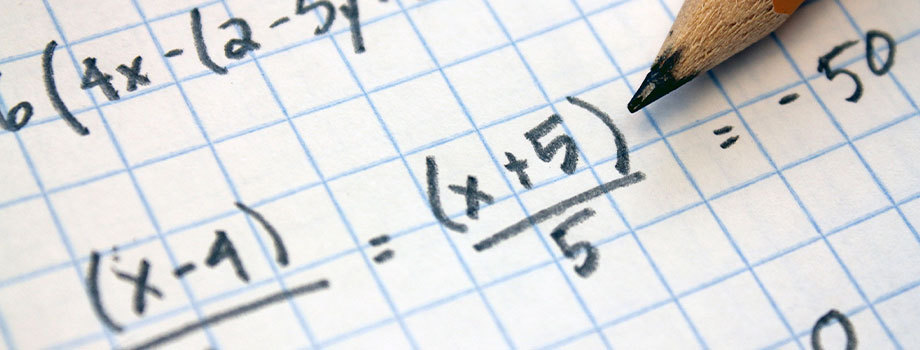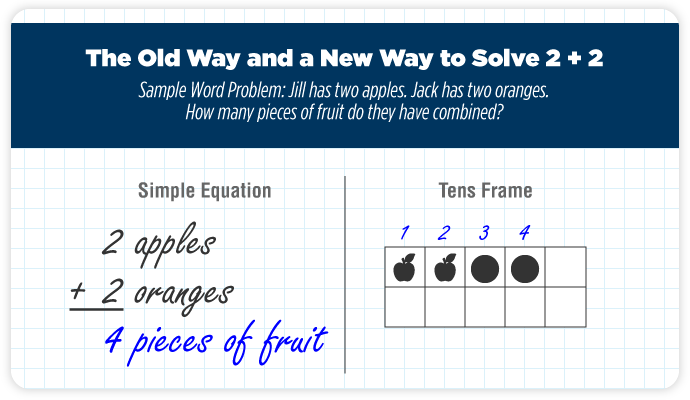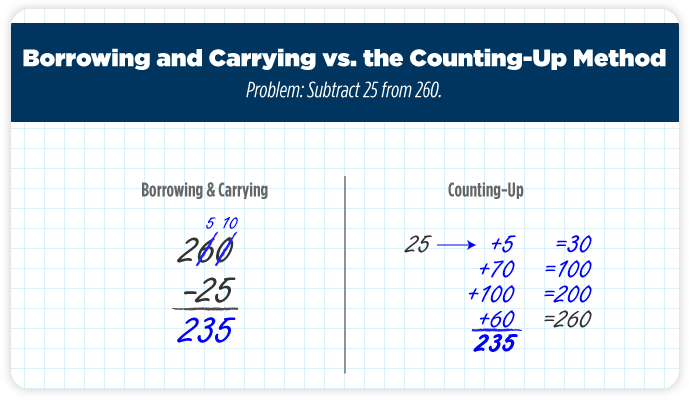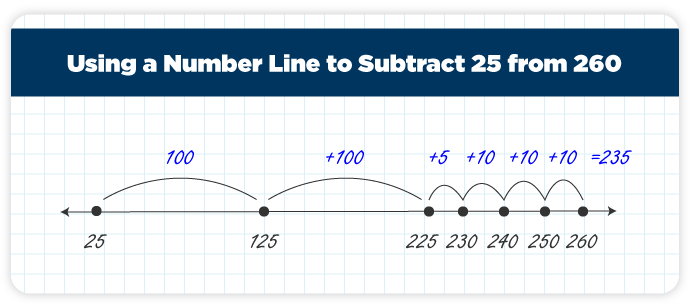# Have Common Core Standards Changed Mathematics?## What Is — and Isn’t — Different in Today’s K-12 Math Classrooms

It’s common practice for mathematics teachers to provide this three-word instruction when they hand out tests: “show your work.” It’s how they check if students have understood concepts that were covered in class. That said, Common Core math standards are changing what it means for students to show their work.

Students in Common Core states don’t learn one way to solve equations. They explore multiple methods, and many are pretty different from the ones their parents were taught in school. To those parents, it seems as though schools are changing the rules of mathematics. That notion found its way to the big screen in “Incredibles 2” when Mr. Incredible struggled to help with his son’s homework.

“Why would they change math?” Mr. Incredible asked. Thankfully, he can rest assured knowing that 2 + 2 still equals 4, even if his son uses a tens frame, as shown below, to find the answer. That’s because Common Core standards don’t actually change the rules of mathematics. They simply require students to solve problems in new ways to hopefully improve their proficiency.Of course, this isn’t the first overhaul of curriculum, nor is it the first time that parents have been confused by key shifts in mathematics instruction. Investigating the evolution of mathematics in public schools can shed light on why the Common Core standards were introduced — and why public acceptance of the standards may be tricky.

## Key Shifts in Mathematics Instruction

Confusion about Common Core math standards reminds some critics of the New Math movement of the 1960s. New Math favored the comprehension of essential concepts instead of memorizing step-by-step procedures. Unfortunately, those concepts were often presented in abstract, theoretical ways that sailed over the heads of young learners.

Why did policymakers think the New Math approach was necessary? An article in TIME points to the Soviet Union’s launch of the satellite Sputnik in 1957. That space-race setback made some politicians fear Americans’ subpar mathematics skills could be the country’s downfall.

The Common Core state standards of 2010 sought to change states’ control of how mathematics was taught. They were developed by state leaders who wanted to provide quality learning to all American students via a single approach to teaching and testing. Under the standards, a student in Illinois would learn the same mathematics concepts on the same schedule as a student in New York and vice versa. Students in both states would also take tests that similarly measured their proficiency.

Are states required to adopt the new standards? No, but generous incentives have encouraged them to do so. The Race to the Top program offered states a share of federal funds worth \$4 billion if they innovated and reformed mathematics in their K-12 schools. One way to score points was to adopt standards such as the ones created by the Common Core initiative. With so much funding at stake, it’s surprising that, according to information from the Common Core State Standards Initiative (CCSSI) at the time of publication, nine states have failed to adopt the standards.

## Features of Common Core Math Instruction

States that adopt Common Core standards must ensure mathematics instruction conforms to three essential principles, as detailed by the CCSSI:

1. Focus on Fewer Concepts: Think quality over quantity. Instead of rushing through a wide range of concepts, students dive into — and master — a narrow set of skills each school year.
2. Maintain Coherence: Students learn concepts that build on each other year after year. Annual curriculum is linked so students connect the dots between what they already know and what they’re learning.
3. Establish Rigorous Standards: Thorough instruction helps students master key concepts and procedures, along with how to apply mathematics lessons to everyday life. Topics are covered in extreme depth to ensure students understand them.

According to CCSSI, grade schoolers should learn three to four concepts each year. A selection of annual topics includes:

• Kindergarten: Count and write whole numbers; identify shapes
• Grade 1: Add and subtract whole numbers (1 to 20); group sets of 10 up to 100
• Grade 3: Multiply and divide whole numbers; explore simple fractions
• Grade 4: Multiply and divide multi-digit numbers; add and subtract simple fractions
• Grade 5: Fluently add and subtract fractions; multiply and divide fractions

When viewed at a high level, the above topics may seem straightforward, but things get tricky when you dig into the nuanced application of the standards. Take a deep breath before you read further — this is when many parents get frustrated.

## Common Core Math Problems

As explained above, students in Common Core schools learn to strengthen their subtraction skills in second grade. They don’t simply learn the old-fashioned method of subtracting one integer from another by borrowing and carrying numbers, though. They use alternate tactics to solve the problem, such as the counting-up subtraction method.

To use this method to subtract 25 from 260, a student would start at the smaller number, 25, and count up to 260 using simple integers, such as this:

1. Add 5 to 25 to get 30 so the ones place is zero for the smaller and larger numbers
2. Count up using numbers divisible by 10: add 70 to 30 to get 100, add another 100 to get 200, and add 60 to get 260
3. Record each count-up amount (5 + 70 + 100 + 60) and total those numbers to find the answer, 235.This can be an effective teaching tool because it is visual. It allows students to see a range of integers that add up to the answer they are calculating. In other words, they start to grasp the logic behind concepts and reasons mathematics procedures work in the real world.

Another helpful visual tool is the number line. It’s a diagram that shows two numbers in relation to each other. To use a number line to solve 260 – 25, students would:

1. Draw a horizontal line
2. Write 25 on the left side of the line and 260 on the right side
3. Make notches for numbers that are sandwiched between 25 and 260, such as 125, 225, 230, 250, and 250
4. Draw hop lines to find the numeric distance between notches
5. Total the amounts above the hop lines to find the answer, 235Is a number line the fastest way to solve a subtraction problem? No, but remember: grade schoolers are just beginning to learn how to add, subtract, multiply, and divide. For them, exploring alternate, or even tedious ways, to solve problems may offer benefits. After all, “Common Core focuses on developing the critical-thinking, problem-solving, and analytical skills students will need to be successful,” the CCSSI said on a webpage dedicated to what parents should know about the standards.